﻿﻿ CI²MA - Investigación

# Full Gallery of Research

## 2015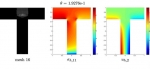29 September 2015: Fundamental Research Areas / Numerical Analysis of Partial Differential Equations
Adaptivity of a mixed method for a nonlinear Brinkman model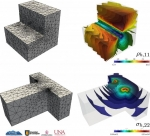24 June 2015: Fundamental Research Areas / Numerical Analysis of Partial Differential Equations
Adaptivity of a pseudostress-based mixed method for 3D linear elasticity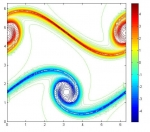23 June 2015: Fundamental Research Areas / Numerical Analysis of Partial Differential Equations
Double shear layer problem with periodic boundary conditions29 March 2015: Fundamental Research Areas / Numerical Analysis of Partial Differential Equations
Secondary clarifier of a wastewater treatment plant27 March 2015: Fundamental Research Areas / Numerical Analysis of Partial Differential Equations
Steady-state regime of a sedimentation-consolidation system25 March 2015: Fundamental Research Areas / Numerical Analysis of Partial Differential Equations
Simulation of Navier-Stokes flows with variable viscosity

## 201426 November 2014: Applied Research Areas / Development of Measurement and Modelating Technics in Hydraulic Engineering
Simulations of the open channel flow with shock waves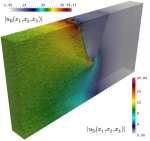19 November 2014: Fundamental Research Areas / Numerical Analysis of Partial Differential Equations
Simulation of flow into a cracked porous medium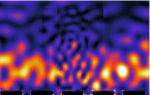28 October 2014: Fundamental Research Areas / Numerical Analysis of Partial Differential Equations
Numerical simulation of light harvesting devices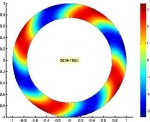27 October 2014: Fundamental Research Areas / Numerical Analysis of Partial Differential Equations
Numerical simulation of the propagation of noise in a moving fluid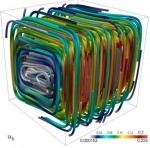20 October 2014: Fundamental Research Areas / Numerical Analysis of Partial Differential Equations
Numerical simulation of a coupled flow-transport problem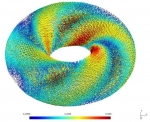18 October 2014: Fundamental Research Areas / Numerical Analysis of Partial Differential Equations
Numerical simulation of the Beltrami field in the torus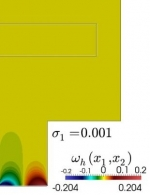15 October 2014: Fundamental Research Areas / Numerical Analysis of Partial Differential Equations
Numerical simulation of the flow in a contracting channel with a porous obstacle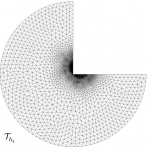10 October 2014: Fundamental Research Areas / Numerical Analysis of Partial Differential Equations
Adaptive numerical solution of the Darcy equations with pressure dependent porosity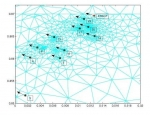9 September 2014: Fundamental Research Areas / Numerical Analysis of Partial Differential Equations
Localization of brain activity from measurements of the electric potential on the surface of the head

 CI²MA, CENTER FOR RESEARCH IN MATHEMATICAL ENGINEERING, UNIVERSIDAD DE CONCEPCIÓN - MAILBOX 160-C, CONCEPCIÓN, CHILE, PHONE: +56-41-2661324